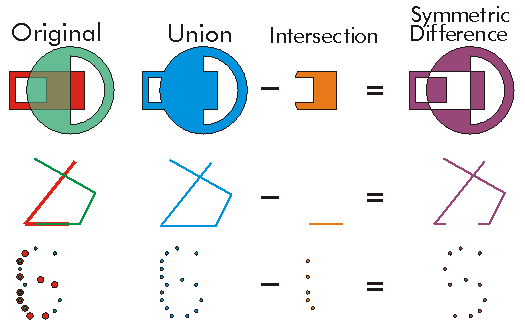This document is archived and information here might be outdated.  Recommended version.

ITopologicalOperator.SymmetricDifference Method (ArcObjects .NET 10.7 SDK)
 ArcObjects Help for .NET developers > ArcObjects Help for .NET developers > ArcObjects namespaces > Geometry > ESRI.ArcGIS.Geometry > Interfaces > IT > ITopologicalOperator Interface > ITopologicalOperator.SymmetricDifference Method
 ArcGIS Developer Help

# ITopologicalOperator.SymmetricDifference Method

Constructs the geometry that contains points from either but not both input geometries.

```[Visual Basic .NET]
Public Function SymmetricDifference ( _
ByVal other As IGeometry _
) As IGeometry
```
```[C#]
public IGeometry SymmetricDifference (
IGeometry other
);
```
```[C++]
HRESULT SymmetricDifference(
IGeometry* other
);
```
```[C++]
Parameters
other
other is a parameter of type IGeometry*
```

#### Product Availability

Available with ArcGIS Engine, ArcGIS Desktop, and ArcGIS Server.

#### Description

The SymmetricDifference between two Geometries of the same Dimension is the Union of those Geometries minus the Intersection of those Geometries.  Thus, the SymmetricDifference is composed only of regions unique to only one of the geometries.

This method does not support GeometryBags.

#### Remarks

SymmetricDifference is basically an XOR between the input geometries.

SymmetricDifference of G1 and G2 can also be described as the Union(Difference(G1, G2), Difference(G2, G1)).

ITopologicalOperator methods must be applied on high-level geometries only. High-Level geometries are point, multipoint, polyline and polygon. To use this method with low-level geometries such as segments (Line, Circular Arc, Elliptic Arc, Bézier Curve), paths or rings, they must be wrapped into high-level geometries types.

This method does not support GeometryBags.[C#]

//The following code shows to wrap a line segment into a polyline in C#

//Assume a line (line1 as ILine) is already created

object obj = Type.Missing;

ISegmentCollection segCollection = new PolylineClass() as ISegmentCollection;

segCollection.AddSegment((ISegment)line1, ref obj, ref obj);

//Set the spatial reference on the new polyline

//The spatial reference is not transfered automatically from the segments

IGeometry geom = segCollection as IGeometry;

geom.SpatialReference = spatialRef;

//Can now be used with ITopologicalOperator methods